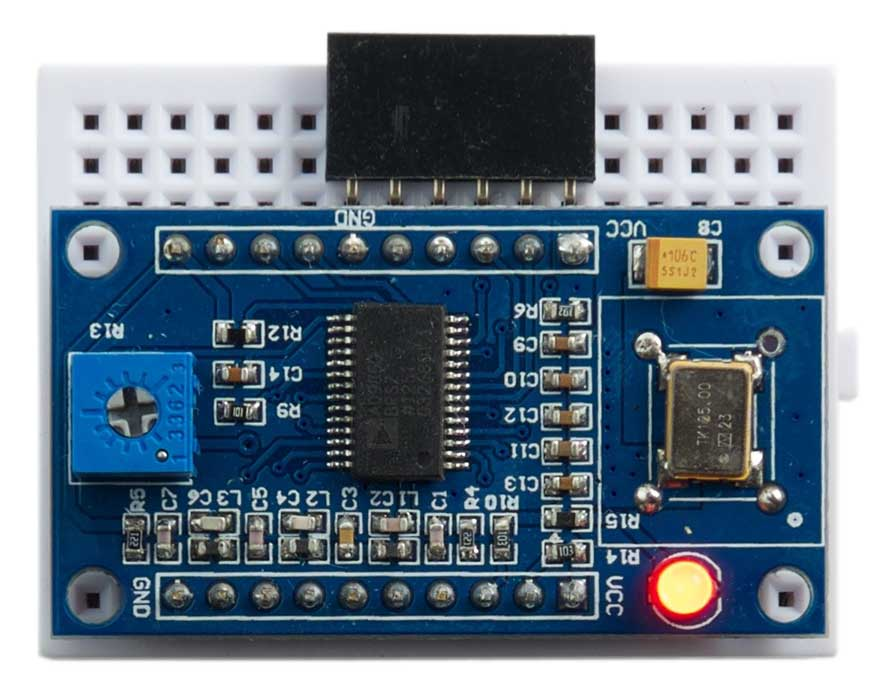## DDS signal generator

This application shows how to build a signal generator capable of generating sine and square waves from below 1 Hz up to above 10 MHz. It's based on the AD9850 DSS Module  which is available from Banggood , AliExpress, or eBay:You control the frequency by writing 32 bits of serial data to its DATA pin. The CLK pin is the clock signal, and the FQ pin is used to update the frequency once the data has been supplied. The RST pin is used to reset the chip. If you're using an Arduino Uno connect the pins as follows:

• +5V to Vcc
• Arduino pin 13 to W_CLK.
• Arduino pin 9 to FQ
• Arduino pin 11 to DAT
• Arduino pin 10 to RST
• GND to GND

A potentiometer on the board allows you to adjust the square wave duty cycle; I found I could only get a square wave with a limited range of settings, so I connected a piezo speaker between Q1 and GND, and adjusted the preset on the board until I got a clearly audible square wave. The sinewave is about 1V p/p, and the square wave is about 5V p/p.

### Initialising the board

Here's the uLisp program ini to initialise the board:

```(defun ini ()
(let ((rst 10)
(clk 13)
(fq 9)
(dat 11))
(pinmode rst t)
(pinmode clk t)
(pinmode fq t)
(pul rst)
(pul clk)
(pul fq)))```

This resets the AD9850 chip, and then sets it into serial mode. It calls this routine pul to pulse a digital output line:

```(defun pul (pin)
(digitalwrite pin t)
(digitalwrite pin nil))```

### Setting the frequency

The following routine sets the frequency to a four-byte value given in the list f, least-significant byte first:

```(defun ton (f)
(with-spi (str 9 2 t)
(mapc (lambda (i) (write-byte i str)) f)
(write-byte #x00 str)))```

To calculate the value f multiply the frequency you want by 4294967296/125000000 = 34.36, where 125000000 is the frequency of the DDS clock crystal, and 4294967296 is 2^32.

For example, to get a frequency of 440 Hz (middle A on the piano) we need a value of 440 x 34.36 = 15118, or #x3b0e in hexadecimal, so the command is:

`(ton '(#x0e #x3b 0 0))`

Using uLisp it's fairly easy to write a program that sweeps the frequency, or adjusts it based on an external input. For example, here's a program to allow you to adjust the frequency using a 10kΩ potentiometer connected between +5V and GND with the wiper connected to analogue input A0:

```(defun dis ()
(loop
(a1 (logand a #xff))
(a2 (ash a -8)))
(with-spi (str 9 2 t)
(mapc (lambda (i) (write-byte i str)) (list 0 a1 a2 0))
(write-byte #x00 str)))
(delay 100)))```

You can vary the frequency in steps of about 34Hz up to about 8kHz.

Here's the whole program: DDS signal generator program.

1. ^ AD9850 datasheet on Analog Devices website.
2. ^ AD9850 DSS Signal generator Module on Banggood.